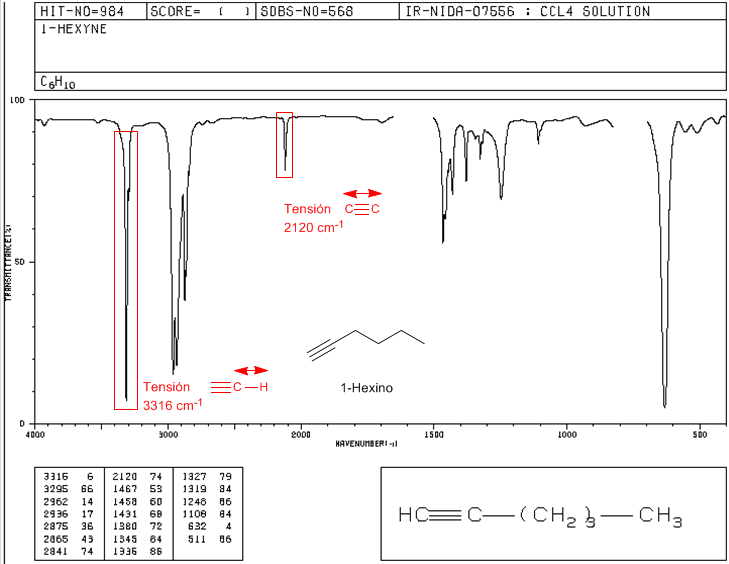♦ Tension $\equiv CH$: 3300 $cm^{-1}$

♦ Tension $-C\equiv C-$: 2150 $cm^{-1}$. Symmetrical alkynes do not present this band, being very weak in the internal ones. The conjugation lowers the value slightly.

IR spectrum of 1-hexineIn the spectrum, in addition to the characteristic bands of the alkyne, the CH tension bands of the chain (below 3000) and the scissor bending bands of CH 2 (1467) and those of symmetric and asymmetric bending of methyl are observed. .

IR spectrum of 2-hexine

2-Hexyne is an internal alkene and does not show the CH$\equiv band at 3300$cm^{-1}$. It also presents a very weak$C\equiv C$voltage band, due to its poor polarity.IR spectrum of phenylacetylene Conjugation lowers the value of the frequency at which the tension band$C\equiv C\$ appears